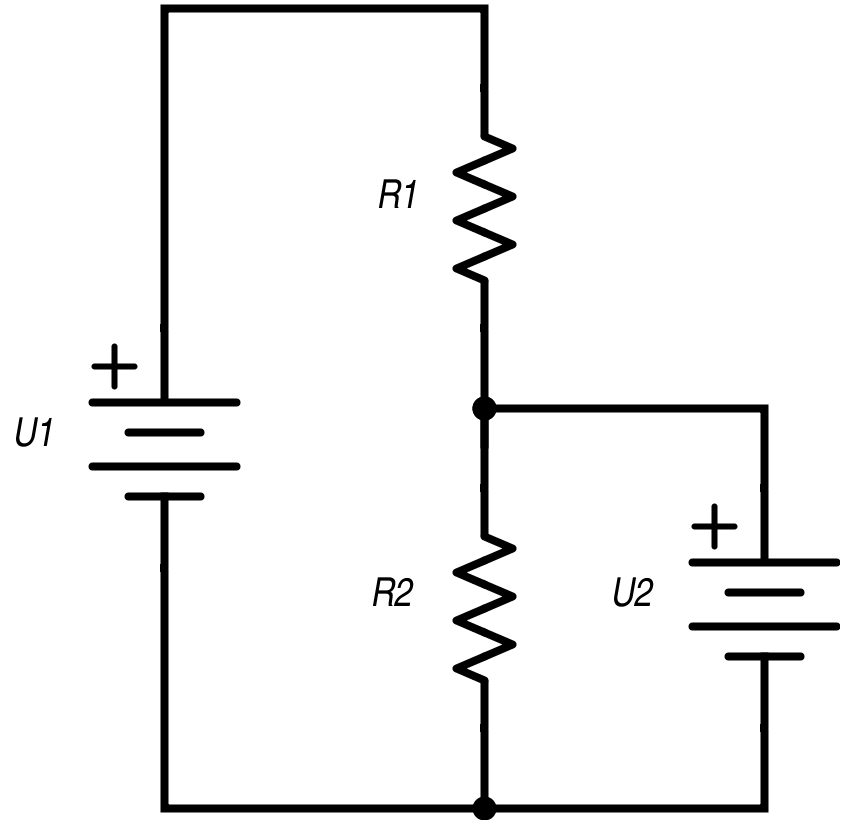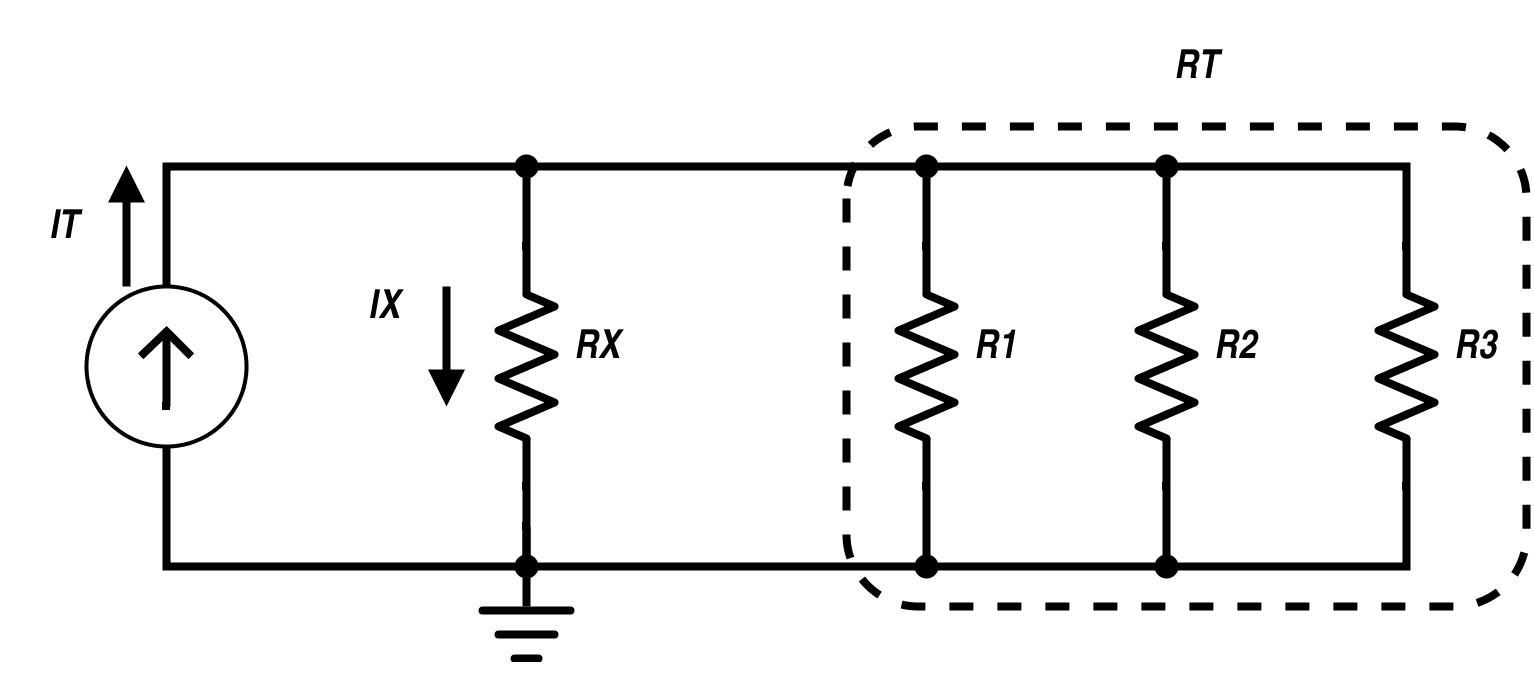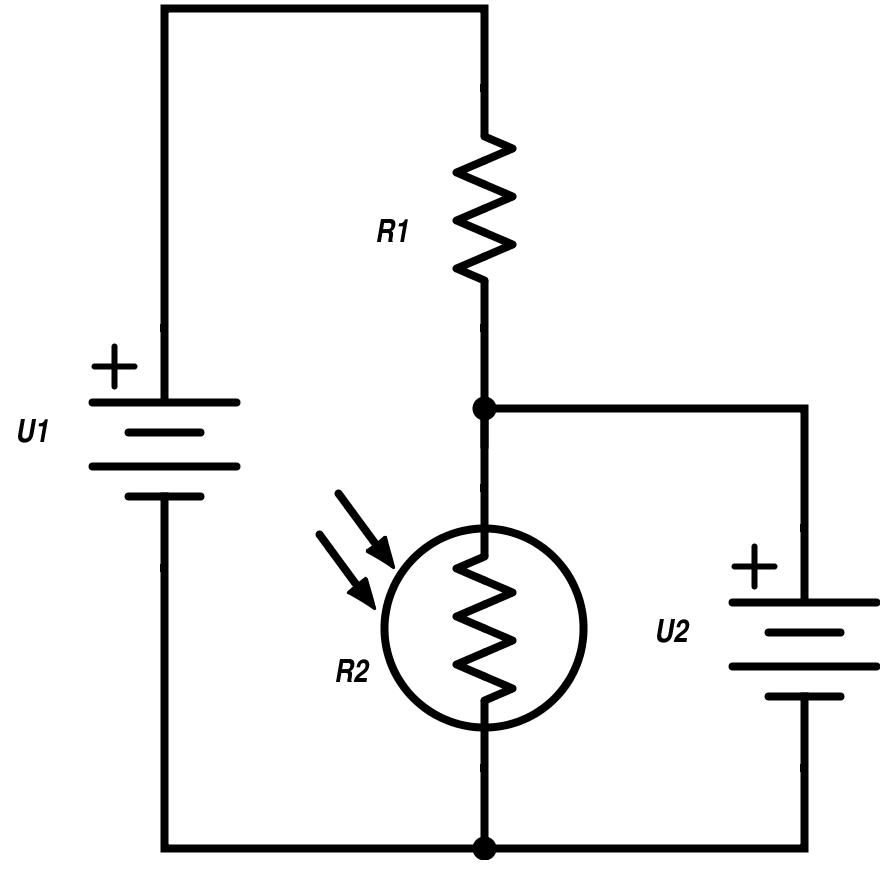Technical Article

# Voltage and Current Dividers: What They Are and What They Do

June 26, 2015 by Tristan Cornell

## This article describes what voltage and current dividers are and how they can be used.

This article describes what voltage and current dividers are and how they can be used.

Beginner

### Kirchoff's Voltage Law

Kirchhoff's Voltage Law states that that the algebraic sum of all the voltages in a loop must equal zero. A practical application of this law is the voltage divider, shown in the figure below.

If U1 is a 9-V battery, R1 a 3-Ohm resistor, and R2 a 6-Ohm resistor, the current flowing in the circuit is

$$\frac{9 \text{ v}}{3 \text{ } \Omega + 6 \text{ } \Omega} = 1 \text{ A}$$.

The voltage across R1 would then be

$$3 \text{ }\Omega \text{ x } 1 \text{ A} = 3\text{ v}$$,

and the voltage across R2 would be

$$6 \text{ }\Omega \text{ x } 1 \text{ A}= 6\text{ v}$$.

The voltage across the individual resistors is equal to the voltage across both resistors times the ratio of the individual resistance to the total resistance. The equation for calculating the voltage across resistor R2, U2, is also shown below.Fig. 1 Voltage Divider Circuit

### Kirchoff's Current Law

Similarly, you can divide currents. The law that governs this is called Kirchoff's Current Law. This law states, "The algebraic sum of all currents entering and exiting a node must equal zero."

To see how this works, refer to the figure below. IT is the total current being supplied to the circuit of four parallel resistors. IX is the current that will flow through RX. As shown by the equation below, the fraction of IT flowing through RX will be equal to IX times the ratio of RX to the total resistance. If all of the resistors are the same value, then the current through each will be the same.

For example, if $$I_{T} = 1 \text{ A}$$ and $$R_{X}=R_{1}=R_{2}=R_{3}=30 \;\Omega$$,

then $$R_{T} = 10 \;\Omega$$ and $$I_{X} = \frac{I_{T}R_{T}}{R_{X}+R_{T}} = \frac{(1)(10)}{30+10} = \frac{10}{40} = 0.25 \text{ A}$$.Fig. 2 Current Divider Circuit

### Applications of Voltage and Current Dividers

Voltage and current dividers have many practical applications. For example, in the circuit shown below, R2 is a photoresistor, which changes value as the intensity of the light impinging on it changes. The voltage across R2, U2, is then a measure of light intensity. You could use this to sense the level of sunlight, or the presence or absence of an object between a light source and the photoresistor. There are, of course, many other applications for voltage and current divider circuits.Fig. 3 Voltage Divider Application

#### $$U_{2}=\frac{U_{1}R_{2}}{R_{1}+R_{2}}$$•Pete Dubler July 27, 2017